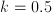# AP Calculus AB : How to find position

## Example Questions

### Example Question #1 : How To Find Position

Given the velocity function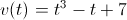, find the position at time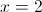with initial position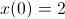.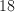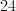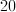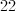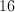Explanation:

First, we find the position function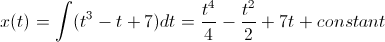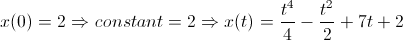Therefore,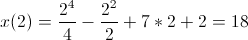.

### Example Question #2 : How To Find Position

Given the acceleration function is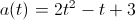, intial velocity is 2 m/s, and the displacement at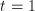is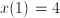.

Find its initial position.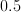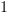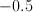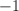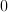Explanation:

First, we find the velocity function by integrating the acceleration function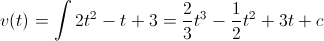Since the initial velocity is 2 m/s, we have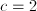.

Then we integrate again to get the displacement function.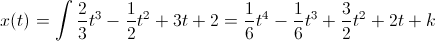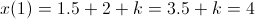This implies